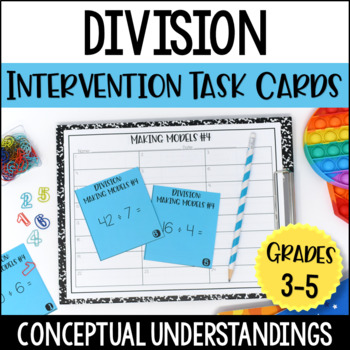# Division Intervention Task Cards | Division Conceptual UnderstandingSubject
Resource Type
Product Rating
File Type

PDF

(1 MB|50+)
Product Description

Students often struggle with division fact fluency and automaticity because they lack a conceptual understanding of division (and how it connects or relates to multiplication). This simple-to-prep and use resource includes over 300 division task cards to help you build that conceptual understanding with your students.

I designed these division task cards to be an extension of my mini-lessons. For example, I will teach a small group lesson on connecting division to multiplication using fact families and then the students will complete the task cards with me, as a math center, with partners, or independently.

This resource includes division task cards for:

•Making Models

•Analyzing Models

•Finishing Models

•Two Types of Division Problems: Group Size Unknown and Size of Group Unknown

•Connecting Multiplication to Division

Here is a detailed list of the conceptual division skills included. Each skill includes a task card set of 24 task cards:

• The students will analyze equal group models and write division problems to match (scaffolded version).
• The students will analyze arrays and write division problems to match (scaffolded version).
• Analyzing arrays and equal group models and write division problems to match
• The students will use small objects or manipulatives to model division situations. (Group Size Unknown)
• The students will use small objects or manipulatives to model division situations. (# of Groups Unknown)
• The students will use small objects or manipulatives to model division situations. (Group Size Unknown and # of Groups Unknown)
• The students will draw a model to represent and solve division problems.
• The students will finish models to solve the division problems. (Group Size Unknown)
• The students will finish models to solve the division problems. (# of Groups Unknown)
• The students will write multiplication problems and division problems to match models.
• The students will use the fact family numbers on triangles to write two multiplication and two division problems.
• The students will write fact families (two multiplication facts and two division facts) for the numbers given.
• The students will solve the division problems. They will then write the related multiplication problems that helped them (or could help them).
• The students will write two division problems that could be solved using multiplication facts shown.

This resource works well for students in grades 3-5 who have received initial instruction on division but struggle to understand the skill conceptually.

Answer keys, direction pages, and recording sheets are included for each task card set.

More Division Resources

FREE Division of the Day for 4th and 5th Grade

FREE Division of the Day for 3rd Grade

Division Resources for Multi-Digit Division

Total Pages
50+
Included
Teaching Duration
1 month
Report this Resource to TpT
Reported resources will be reviewed by our team. Report this resource to let us know if this resource violates TpT’s content guidelines.